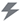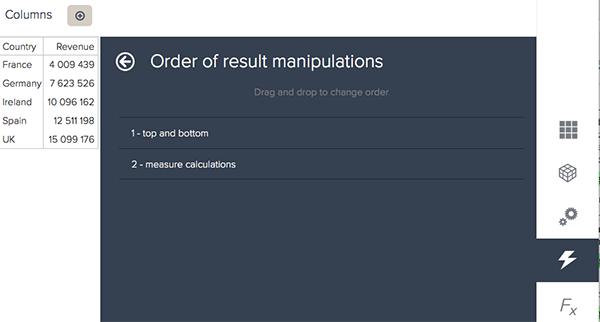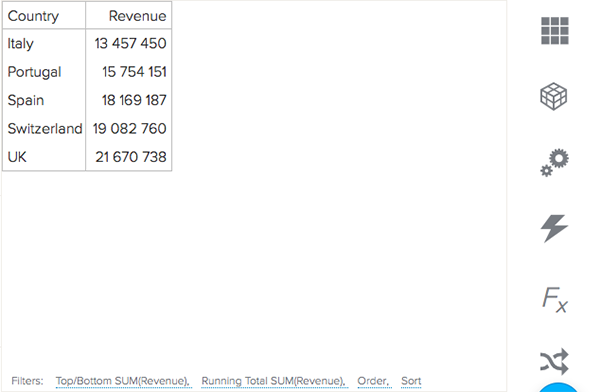Setting the order for your result manipulations

Follow

If you are applying a number of different result manipulations, the order they are calculated is very important. Results will always be computed based on the first result manipulation listed.

For example, if you want to look at the rolling total of the top results in your data, you would first want to calculate the top results and then the rolling total. This would mean your top/bottom filter would be listed before your result path calculation.

To order result manipulations
1. In the Query Builder, click the Result manipulation icon ().
2. Select the Order of result manipulation option.
3. Drag and drop the manipulations to your preferred order. For example, if you would like to look at the rolling total for the top results in your data, you would first compute the top results and then the rolling total. This means your top and bottom manipulation would be listed before your metric calculations.4. Click Apply.